amortization.com Ltd.
Burlington, Ontario

905-639-0374
905-407-7988

info@amortization.comBlended Rates

QUESTION: A lender offers a mortgage at 5.95% for the first year and 7.15% for the remaining four years or a blended rate of 6.85% for 5 years. Which is the better option?

Let us first generate an amortization schedule based upon the 5.95% rate, then change the rate inside the SPREADSHEET schedule. Assume it to be \$100,000 amortized for 25 years and we will use semi-annual compounding as this example is taken directly from a Canadian lenders brochure. We will also assume a constant cash flow meaning that the monthly payment remains the same over the 60 months. Some lenders recalculate and change the monthly payment at each rate change so it is always wise to ask the lender which method they use.

(Screenshot 1)

We now change the rate from 5.95% for the last four years to 7.15 % as shown below;

(Screenshot 2)

NOTE: the rate change for the 13th payment is entered at the 12th payment because as soon as the 12th payment is made the rate is immediately changed, that is how the MORTGAGE2 PRO amortization spreadsheet is designed to work. The constant, 360 day monthly interest factor for an AIR of 5.95% with semi-annual compounding is

(Screenshot 3)

0.004897965062376 x 98,342.33 = 481.68 (green)

(Screenshot 4)

The balance owing (at Pmt #60 in green) to the lender after 5 years is \$94,852.89 using 5.95% for year one and 7.15% for the next four years. One might be tempted now to just guess at a new rate until the balance after 60 payments is the same (\$94,852.89), and that would be the blended rate. It would be nice if it was that simple, but it is not.

Lenders reinvest your monthly payment each month (deemed reinvestment) in order to achieve the yield on your mortgage. For example a mortgage at a nominal rate (nominal rates are also called annual interest rates, AIR) of 12% using semi-annual compounding actually gives the lender a 12.36% effective interest rate (EIR). For every nominal rate quoted there is a corresponding effective interest rate due to the type of compounding chosen. If the nominal rate is annually compounded then the effective interest rate is equal to the nominal rate, thus a yearly basis is always implied when one speaks of an effective interest rate. A mathematical "trick" that works only for mortgages that have semi-annual compounding allows one to quickly figure out an EIR from an AIR is to divide the nominal rate by two, shift the decimal place two places to the left, square the result, shift the decimal place two places to the left again and add it to the initial nominal rate;

AIR=12% EIR=12.36%

12.0/2 =6 6x6=36 .36

12.0 +.36 = 12.36%

One of the unique features of MORTGAGE2 PRO and MORTGAGE2 PRO PRO is the ability to to perform a present value /future value calculation on a cash flow and show what it accumulates to in the future. This is exactly what the lender does. Thus put all of the 60 payments equal to zero and the cash flow of \$636.84 to the lender will accumulate to \$140,443.98 if the lender reinvests the payment received each month.

(Screenshot 5)

You now need a simple PV/FV calculator or using the one in MORTGAGE2 PRO

(Screenshot 6)

that allows one to do a PV/FV calculation as shown below;

(Screenshot 7)

The 7.028792 % from the PV/FV calculator is the effective interest rate, EIR. To get the corresponding AIR, using the CALCULATOR, take a few guess' at an AIR, and recalculate on any variable except the AIR, until you get the EIR to come close to 7.029

(Screenshot 8)

The 6.91% (which corresponds to the EIR of 7.0293703) is the correct blended rate that is equivalent to year one at 5.95% and the next four years at 7.15%.

ANSWER: The lender offering a blended rate of 6.85%, is actually giving borrowers a bit of deal! Below is proof that 6.91% is the correct blended rate. That same cash flow appreciating at 6.91% (deemed reinvestment) for the next 60 months accumulates to \$140,447.77

(Screenshot 9)

which is within \$3.79 of the initial calculation. The difference is because the outcome is very sensitive to the number of decimal places used.

It should be mentioned that you can also use a 365 day year in this analysis. North American lenders use both methods for monthly payment mortgages for various reasons. Below is the exact analysis as above,

except using a 365 Days per Year basis, which is called an exact day monthly payment mortgage in the industry.

(Screenshot 10)

You will notice after 60 payments (12 payments @ 5.95% and the remaining 48 payments @ 7.15%) that the borrower owes the lender approximately 20 dollars more using this method (\$94,872.22 - \$94,852.89 = \$19.33).

The lender is making a "little" more via the 365 day method. A complete analysis shows that the blended interest rate is greater in the third decimal place, and thus quoting rates to 2 decimal places hides this fact. The purpose of the exercise is to demonstrate the method used to verify the blended rate figure within reasonable limits. As mentioned above the deemed reinvestment method is very sensitive to the number of decimal places used in the calculation. Here is an area where standardization would be in order at least in North America.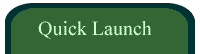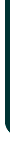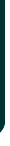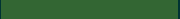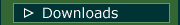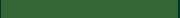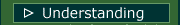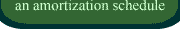VIDEOSamortizationdotcom Mortgage Calculator for iPhone

Introduction to Canadian and American Mortgages

Seminar on prepaying principal (Part A)

Seminar on prepaying principal (Part B)

Global TV Interview regarding 40 Year MortgagesLook for this logo on the Apple Store!

 < Go Back